Next: Detached Shocks Up: Two-Dimensional Compressible Inviscid Flow Previous: Supersonic Compression by Turning

# Supersonic Expansion by Turning

Up to now, we have only considered turns that are concave. That is, turns in which the wall is deflected into the oncoming flow. Let us now consider what happens in a convex turn, where the wall is deflected away from the oncoming flow. In particular, let us investigate supersonic flow over a convex corner, such as that illustrated in Figure 15.8(a).

A convex turn through a single oblique shock, like that illustrated in Figure 15.8(a), is not possible. In such a turn, the deviation of the flow, as it passes through shock, is inevitably away from the plane of the shock front. Such a deviation would require the downstream normal flow speed to exceed the upstream normal flow speed (because the upstream and downstream tangential flow speeds are equal). (See Section 15.2.) However, although this would satisfy the equations of fluid motion, it would lead to a spontaneous decrease in specific entropy across the shock front, and is, thus, forbidden by the second law of thermodynamics. (See Section 14.8.)

What actually happens is as follows. The nonlinear mechanism that tends to steepen a compression (see Sections 14.4 and 15.5) produces the opposite effect in an expansion. In particular, instead of being convergent, the Mach lines are divergent, as shown in Figure 15.8(b) and (c). Consequently, there is a tendency for velocity gradients to decrease with increasing distance from the wall. Thus, an expansion is isentropic throughout the fluid.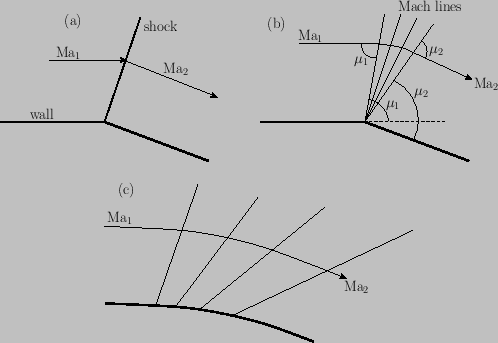The expansion at a corner takes place via a fan of straight Mach lines that is generally known as a Prandtl-Mayer expansion fan. [See Figure 15.8(b).] The arguments that lead to this conclusion are as follows. First, the flow up to the corner is uniform, at Mach number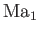(say), and, thus, the leading Mach line must be straight, and inclined to the flow at the Mach angle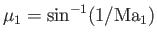. Because of the limited upstream influence in supersonic flow, the same argument may be applied to each succeeding portion of the flow. The terminating Mach line stands at the angle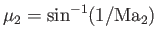to the wall. Here,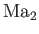is the Mach number of the downstream flow. Second, because there is no characteristic length to define a scale in this problem, any variation of flow parameters can only be with respect to angular position, measured relative to the corner. That is, flow conditions must be constant along rays emanating from the corner.

Figure 15.8(c) shows a typical expansion over a continuous convex turn. Because the flow is isentropic, it is also reversible (i.e., if the direction of the flow is reversed then the fluid is isentropicaly compressed).

Equation (15.35), which specifies the relationship betweenand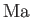in an isentropic compression or expansion by turning, can be written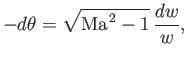(15.36)

or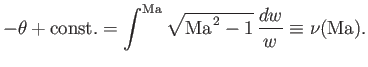(15.37)

In order to evaluate the integral, and, thus, derive an explicit form for the function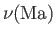, we need to rewritein terms ofusing the definition(15.38)

Here,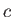is the local sound speed. Given that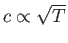[see Equation (14.45)], it follows from Equation (14.58) that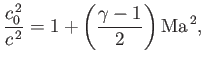(15.39)

whereis the stagnation sound speed. Thus,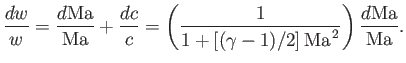(15.40)

The function, which is known as the Prandtl-Mayer function, is then given by (see Exercise x)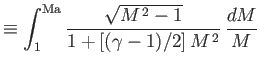(15.41)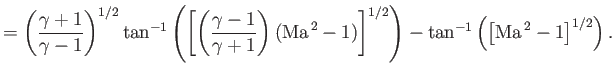Here, the constant of integration has been arbitrarily chosen such that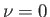corresponds to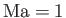. A supersonic Mach number,, is thus associated with a definite value of the function. In fact, asincreases from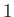to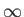,increases monotonically from 0 to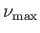, where(15.42)

Equation (15.37) yields(15.43)

as the relationship between the deflection angle,, and the Mach number,, in an isentropic compression or expansion by turning. In a compressive turn,decreases, whereas, in an expansive turn, it increases: in each case, by an amount equal to the change in. Here, we have set, because only the change inmatters. Note thatis positive in compressive turns, and negative in expansive turns.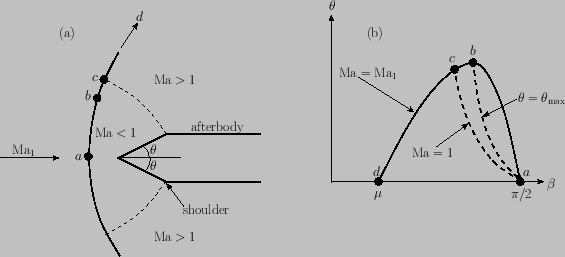Next: Detached Shocks Up: Two-Dimensional Compressible Inviscid Flow Previous: Supersonic Compression by Turning
Richard Fitzpatrick 2016-03-31